# Oscillations and Waves Flashcards Preview

## IB SL Physics > Oscillations and Waves > Flashcards

Flashcards in Oscillations and Waves Deck (67):
1

2

## A mass is suspended from a vertical spring. It is set into oscillation by pulling it down a short distance and then releasing it. At which one of the labelled points, during the subsequent motion, will the acceleration be zero?3

4

## A ray of light travelling in glass is incident on the glass-air boundary at an angle pheta to the normal as shown in the diagram. If pheta is greater than the critical angle which one of the rays best shows the path of the ray?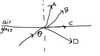5

6

## The diagram shows two loudspeakers connected to the same signal generator. As a microphone is moved along the path XY a series of maximum and minimum sound levels is detected.Which one of the following actions on its own will increase the separation between the maximum and minimum sound levels? A. Moving the speakers closer to the line XY. B. Increasing the separation between the speakers. C. Increasing the amplitude of the sound waves. D. Decreasing the frequency of the sound waves.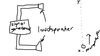7

8

## The diagram represents, at a particular instant of time, a transverse wave travelling to the right along a rope. One section of rope has been marked by tying a ribbon around it at point P.9

10

## Monochromatic light incident on two narrow parallel slits produces a fringe pattern on a screen, with the intensity distribution shown below.If the same slits were spaced further apart, which of the following diagrams best shows the intensity distribution pattern that would result?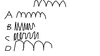11

## The diagram below shows ripples on the surface of water at one instant of time. The ripples are moving right to left and a small object, P, is floating in the water. After a quarter of a time period, which letter correctly shows the position of the floating object?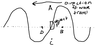12

13

## Two waves of equal frequency, wavelength and amplitude, A, are travelling along a string towards the same point P as shown below.If the waves arrive at P at the same time, which of the following best describes the subsequent motion of the string at point P? A. It will not move. B. It will oscillate with amplitude A. C. It will oscillate with amplitude 2 A. D. It will oscillate with varying amplitude.14

## An organ pipe is closed at one end and open at the other as shown below.Which one of the following diagrams correctly shows the positions of the displacement nodes and antinodes when the fundamental standing wave is formed in the tube?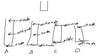15

## Water waves at the surface of a pond pass a floating log of length L. The log is at rest relative to the bank. The diagram shows wave crests at one instant.The number of crests passing the log per unit time is N. The speed of the water waves relative to the log at rest is A. (L/7)(N-1)B. (L/6)(N-1)C. (L/7)ND. (L/6)N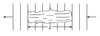16

## Two identical triangular pulses of amplitude X travel toward each other along a string. At the instant shown on the diagram below, point M is midway between the two pulses. The amplitude of the disturbance in the string as the pulses move through M is A. 2X. B. X. C. X/2 D. 0.17

## A person is walking along one side of a building and a car is driving along another side of the building.The person can hear the car approach but cannot see it. This is explained by the fact that sound waves A. travel more slowly than light waves. B. are diffracted more at the corner of the building than light waves. C. are refracted more at the corner of the building than light waves. D. are longitudinal waves.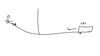18

## A pulse is sent down a string fixed at one end. Which one of the following diagrams best represents the reflected pulse?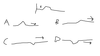19

20

## The displacement d of a particle in a wave varies with distance x along a wave and with time t as shown below.Which expression gives the speed of the wave?A.  l/4rB. l/2rC. l/rD. 2l/r21

## A plane wave approaches and passes through the boundary between two media. The speed of the wave in medium 1 is greater than that in medium 2. Which one of the following diagrams correctly shows the wavefronts?22

## Two particles X and Y are situated a distance (1/2)λ apart on a stationary wave of wavelength λ. The variation with time t of the displacement of X is shown below.Which one of the following correctly shows the variation with time t of the displacement of particle Y?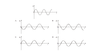23

## The diagram shows the variation with distance x along a wave with its displacement d. The wave is travelling in the direction shown.The period of the wave is T. Which one of the following diagrams shows the displacement of the wave at a time T/4 later?24

25

26

## Jeremy is walking alongside a building and is approaching a road junction. A fire engine is sounding its siren and approaching the road along which Jeremy is walking. Jeremy cannot see the fire engine but he can hear the siren. This is due mainly to A. reflection. B. refraction. C. the Doppler effect. D. diffraction.27

## On which one of the following graphs is the wavelength and the amplitude a of a wave correctly represented?28

29

30

## The diagram below shows two wave pulses moving towards one another.Which one of the following diagrams shows the resultant pulse when the two pulses are superposed?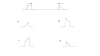31

32

## The variation with time t of the separate displacements d of a point in a medium due to two waves are shown below.The waves are superposed. Which of the following diagrams shows the variation with time t of the resultant displacement d of the point in the medium?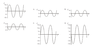33

## A loudspeaker emits sound of frequency f. The sound waves are reflected from a wall. The arrangement is shown below.When a microphone is moved along the line SW, minimum loudness of sound is detected at points P, Q and R. There are no other minima between these points. The separation of the minima is d. The speed of the sound wave isA. (1/2) fdB. f/dC. fdD. 2fd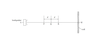34

35

36

37

## The graph below shows the variation of air pressure with distance along a wave at one given time. The arrow indicates the direction of travel of the wave.The air pressure at point P is  A. increasing.  B. decreasing.  C. constant.  D. zero.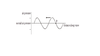38

## Sound waves move faster in warm air than in cold air. The diagram below shows plane waves in cold air moving towards a boundary with warm air.Which of the arrows shows the possible direction of waves after reaching the boundary?  A. I  B. II  C. III  D. IV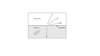39

40

41

## Diagram 1 below shows the displacement of part of a medium through which a wave is travelling at time t = 0. Diagram 2 shows the displacement at a later time t = 4.0 s in which the wave has moved forward 10 cm. In this time, the point P on the wave has moved from a crest through zero displacement to a troughThe wavelength of the wave is  A. 5.0 cm.  B. 10 cm.  C. 20 cm.  D. 40 cm.42

## The diagram below shows a pulse travelling along a rope from X to Y. The end Y of the rope is tied to a fixed supportWhen the pulse reaches end Y it will  A. disappear.  B. cause the end of the rope at Y to oscillate up and down.  C. be reflected and be inverted.  D. be reflected and not be inverted.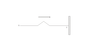43

44

45

## A source produces water waves of frequency 10 Hz. The graph shows the variation with horizontal position of the vertical displacement of the surface of water at one instant in time.The speed of the water waves is  A. 0.20 cm s−1 .  B. 4.0 cm s−1 .  C. 10 cm s−1 .  D. 20 cm s−1 .46

47

## A bat approaches an insect of wing span length d. The bat emits a sound wave. The bat detects the insect if the sound is reflected from the insect.The insect will not be located if  A. the insect’s speed is less than the speed of the sound wave.  B. the insect’s wing beat frequency is greater than the frequency of the sound wave.  C. the length d is much greater than the wavelength of the sound wave.  D. the length d is much smaller than the wavelength of the sound wave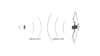48

49

## The diagram below shows a transverse wave on a string. The wave is moving from right to left.In the position shown, point X has zero displacement and point Y is at a position of maximum displacement. Which one of the following gives the subsequent direction of motion of point X and of point Y?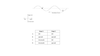50

## Light is incident on an air-glass boundary as shown below.Which one of the following is a correct statement of Snell’s law?  A. sinP= constant × sinR  B. sinP= constant × sinS  C. sinQ= constant × sinR  D. sinQ= constant × sinS51

52

## A    string    is    held    horizontally    with    one    end    attached    to    a    fixed    support.        Two    pulses    are    created    at    the    free    end    of    the    string.        The    pulses    are    moving    towards    the    fixed    support    as    shown    in    the    diagram     below.Which one of the following diagrams is a possible subsequent picture of the string?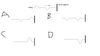53

## A water surface wave (ripple) is travelling to the right on the surface of a lake. The wave has period T. The diagram below shows the surface of the lake at a particular instant of time. A piece of cork is floating    in    the    water    in    the    position    shown. Which is the correct position of the cork a time T/4 later?54

## The diagram below shows a photograph of part of a standing wave    on    a    string    that    is    fixed    at    both    ends. The wavelength of the wave is 40 cm. At a particular time the point marked P has displacement + 2.0 cm and moves with velocity + 80 m s–1.A second point Q on the string is at a distance of 20cm from P. At the same time that the photograph above was taken, the displacement and velocity of point Q were     displacement velocity A. + 2.0 cm + 80 m s–1 B. + 2.0 cm – 80 m s–1 C. – 2.0 cm + 80 m s–1 D. – 2.0 cm – 80 m s–155

## A source of sound is placed near the open end of a cylindrical tube that lies on a horizontal table. The tube has some powder sprinkled along its length. The powder collects in piles along the length of the tube as shown below.The distance between two consecutive piles of powder is d and the speed of sound in the tube is v. The frequency of the source isA. v/2dB. v/dC. dvD. 2dv56

## A wave is travelling through a medium. The diagram shows the variation with time t of the displacement d of a particle of the medium from t = 0 to t = 25 ms.Which of the following correctly gives the frequency and the amplitude of the wave?frequency / hz         amplitude / cm A. 2.0 x 10–2        2.0 B. 2.0 x 10–2         1.0 C. 50                  2.0 D. 50               1.0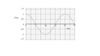57

## Waves of frequency f travel with speed c in air and enter a medium M of refractive index 1.5. Which of the following correctly gives the frequency and speed of the waves in the medium M?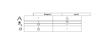58

59

## Plane wavefronts are incident on a barrier as shown below. Which of the following best shows the shape of the wavefronts on the other side of the barrier?60

61

## Which of the following diagrams best shows the path of a ray of monochromatic light through a glass prism in air?62

## Plane parallel wavefronts are incident on an obstacle. Which of the following diagrams best shows the diffraction of the waves around the obstacle?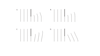63

## A vibrating tuning fork is held above the top of a tube that is filled with water. The water gradually runs out of the tube until a maximum loudness of sound is heard. Which of the following best shows the standing wave pattern set up in the tube at this position?64

65

## The diagram below shows plane wavefronts of a wave that is approaching the boundary between two media, X and Y. The speed of the wave is greater in medium X than in medium Y. The wave crosses the boundary.66

## The graph below shows the variation with time t of the separate displacements d of a medium, at a particular point in the medium due to two waves, P and Q.The amplitude of the wave resulting from the interference of P and Q is  A. 0.0 mm.  B. 1.0 mm.  C. 1.4 mm.  D. 2.0 mm.67